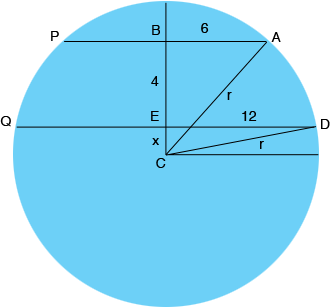SEARCH HOMEMath Central Quandaries & QueriesQuestion from Raj, a student: AB and CD are two chords which are in the same side of the circle.The length of AB=12 and CD= 24.if the distance between them is 4 then find their radius. Pls help me I just believe on you guys I am having my exam after 3 daysHi Raj,

I drew a diagram.Use Pythagoras' theorem and triangle $DEC$ to write a realtionship between $r$ and $x.$

Use Pythagoras' theorem and triangle $ABC$ to write a realtionship between $r$ and $x.$

Solve for $x.$

PennyMath Central is supported by the University of Regina and The Pacific Institute for the Mathematical Sciences.